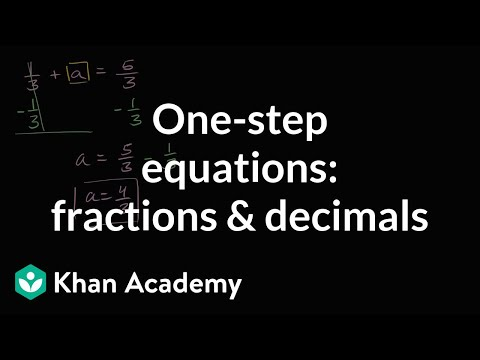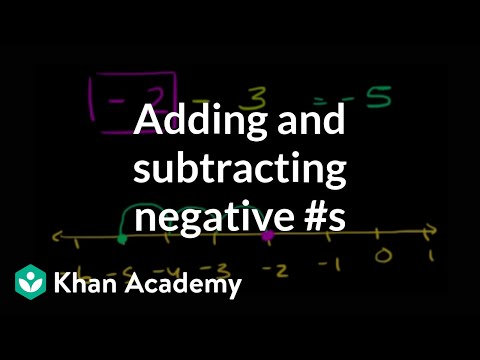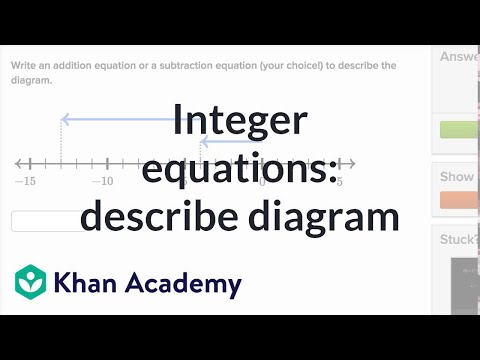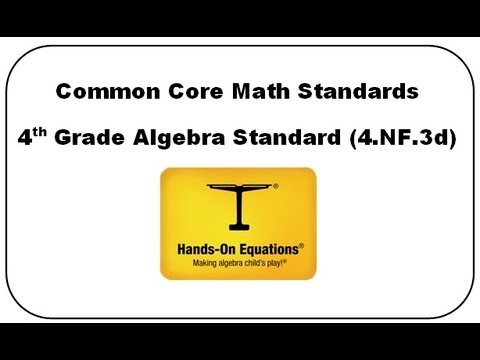# One-step equations with addition and subtraction

##By Khan Academy

Learn how to solve one-step addition and subtraction equations by adding or subtracting the same thing from both sides of the equation.# One-step addition and subtraction equations with fractions and decimals

##By Khan Academy

Learn how to solve one-step addition and subtraction equations by adding or subtracting the same thing from both sides of the equation.# Solving One Step Equations By Addition and Subtraction

##By Mathispower4u

Video about solving one step equations by addition and subtraction.# One-step equations with addition and subtraction

##By Khan Academy

Some quick examples to practice solving a variety of one step equations. All 4 operations (add, subtract, multiple, divide) are paired with variables.# Solving One Step Equations By Addition and Subtraction

##By Mathispower4u

Video about solving one step equations by addition and subtraction.# One-Step Subtraction Equations - MathHelp.com - Math Help

##By yourteachermathhelp

This video takes you through a one step subtraction equation and also shows you how to check your answer.# One-step addition and subtraction equations with fractions and decimals

##By Khan Academy

Learn how to solve one-step addition and subtraction equations that have fractions and decimals in them.# One-step addition and subtraction equations with fractions and decimals

##By Khan Academy

Some quick examples to practice solving a variety of one step equations. All 4 operations (add, subtract, multiple, divide) are paired with variables.# One-step equations with addition and subtraction

##By Khan Academy

Learn how to solve one-step addition and subtraction equations by adding or subtracting the same thing from both sides of the equation.# Ex: Solve One Step Equations With Fraction by Adding or Subtracting

##By Mathispower4u

This video provides two examples of how to solve a one step linear equation by adding and subtracting.# One-step equations with addition and subtraction

##By Khan Academy

Some quick examples to practice solving a variety of one step equations. All 4 operations (add, subtract, multiple, divide) are paired with variables.# One-step equations with addition and subtraction

##By Khan Academy

Learn how to solve this equation: a + 5 = 54ï¿½ï¿½ï¿½ï¿½ï¿½ï¿½ï¿½ï¿½ï¿½ï¿½ï¿½ï¿½# Integer addition and subtraction: equations and number lines

##By Khan Academy

Learn how to add and subtract negative numbers. The problems solved in this video are 2 - 3 = -1 and -2 - 3 = -5 and -2 + 3 = 1 and 2 - (-3) = 5 and -2 - (-3).# Integer addition and subtraction: equations and number lines

##By Khan Academy

Integer equations to describe diagram# One-step addition and subtraction equations with fractions and decimals

##By Khan Academy

Learn how to solve this equation: a + 5 = 54ï¿½ï¿½ï¿½ï¿½ï¿½ï¿½ï¿½ï¿½ï¿½ï¿½ï¿½ï¿½# One-step equations with addition and subtraction

##By Khan Academy

Learn how to solve this equation: a + 5 = 54ï¿½ï¿½ï¿½ï¿½ï¿½ï¿½ï¿½ï¿½ï¿½ï¿½ï¿½ï¿½# Algebra for 4th Graders:CCSSM 4.NF.3d

##By Henry Borenson

Use visual models and equations to represent and solve word problems involving addition and subtraction of fractions (4.NF.3d)# Integer addition and subtraction: equations and number lines

##By Khan Academy

Learn how to add and subtract negative numbers. The problems solved in this video are 2 - 3 = -1 and -2 - 3 = -5 and -2 + 3 = 1 and 2 - (-3) = 5 and -2 - (-3).# Integer addition and subtraction: equations and number lines

##By Khan Academy

Learn how to add and subtract negative numbers. The problems solved in this video are 2 - 3 = -1 and -2 - 3 = -5 and -2 + 3 = 1 and 2 - (-3) = 5 and -2 - (-3).# Integer addition and subtraction: equations and number lines

##By Khan Academy

Learn how to add and subtract negative numbers. The problems solved in this video are 2 - 3 = -1 and -2 - 3 = -5 and -2 + 3 = 1 and 2 - (-3) = 5 and -2 - (-3).